# Maharashtra Board 11th Commerce Maths Solutions Chapter 8 Continuity Ex 8.1

Balbharati Maharashtra State Board 11th Commerce Maths Solution Book Pdf Chapter 8 Continuity Ex 8.1 Questions and Answers.

## Maharashtra State Board 11th Commerce Maths Solutions Chapter 8 Continuity Ex 8.1

Question 1.
Examine the continuity of
(i) f(x) = x3 + 2x2 – x – 2 at x = -2
Solution:
f(x) = x3 + 2x2 – x – 2
Here f(x) is a polynomial function and hence it is continuous for all x ∈ R.
∴ f(x) is continuous at x = -2

(ii) f(x) = $$\frac{x^{2}-9}{x-3}$$ on R
Solution:
f(x) = $$\frac{x^{2}-9}{x-3}$$; x ∈ R
f(x) is a rational function and is continuous for all x ∈ R, except at the points where denominator becomes zero.
Here, denominator x – 3 = 0 when x = 3.
∴ Function f is continuous for all x ∈ R, except at x = 3, where it is not defined.Question 2.
Examine whether the function is continuous at the points indicated against them.
(i) f(x) = x3 – 2x + 1, for x ≤ 2
= 3x – 2, for x > 2, at x = 2
Solution: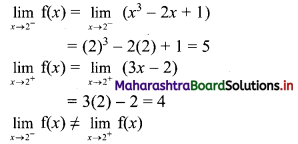∴ Function f is discontinuous at x = 2

(ii) f(x) = $$\frac{x^{2}+18 x-19}{x-1}$$ for x ≠ 1
= 20, for x = 1, at x = 1
Solution: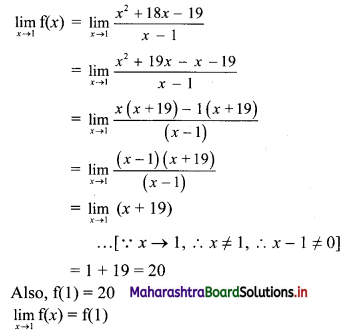∴ f(x) is continuous at x = 1

Question 3.
Test the continuity of the following functions at the points indicated against them.
(i) f(x) = $$\frac{\sqrt{x-1}-(x-1)^{\frac{1}{3}}}{x-2}$$ for x ≠ 2
= $$\frac{1}{5}$$ for x = 2, at x = 2
Solution: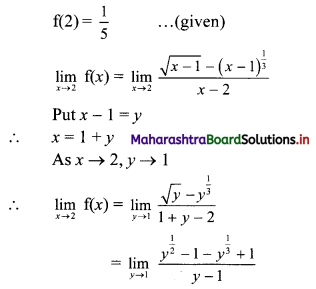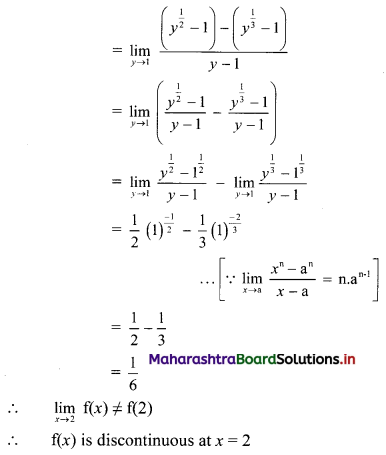(ii) f(x) = $$\frac{x^{3}-8}{\sqrt{x+2}-\sqrt{3 x-2}}$$ for x ≠ 2
= -24 for x = 2, at x = 2
Solution: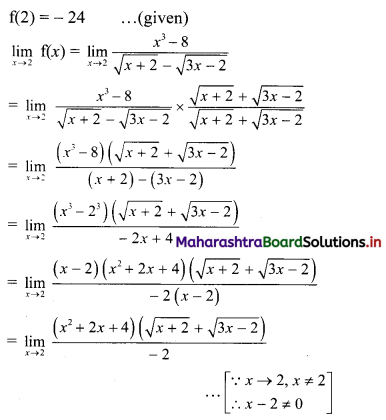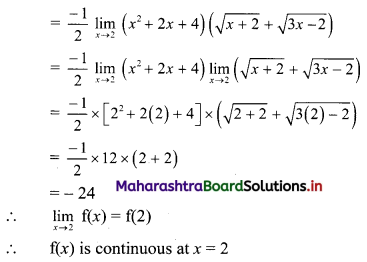(iii) f(x) = 4x + 1 for x ≤ $$\frac{8}{3}$$
= $$\frac{59-9 x}{3}$$, for x > $$\frac{8}{3}$$, at x = $$\frac{8}{3}$$
Solution: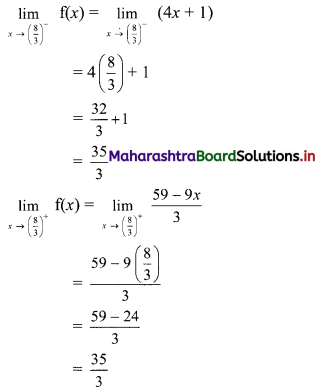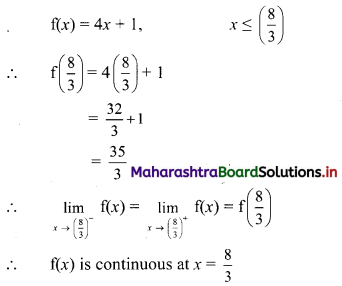(iv) f(x) = $$\frac{x^{3}-27}{x^{2}-9}$$ for 0 ≤ x < 3
= $$\frac{9}{2}$$, for 3 ≤ x ≤ 6, at x = 3
Solution: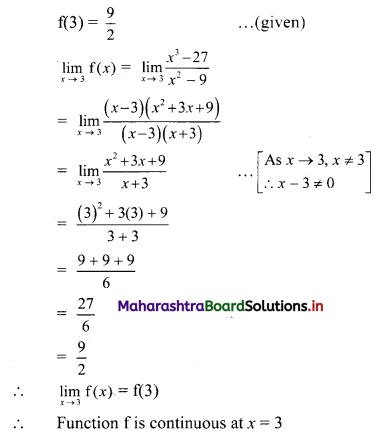Question 4.
(i) If f(x) = $$\frac{24^{x}-8^{x}-3^{x}+1}{12^{x}-4^{x}-3^{x}+1}$$, for x ≠ 0
= k, for x = 0
is continuous at x = 0, find k.
Solution:
Function f is continuous at x = 0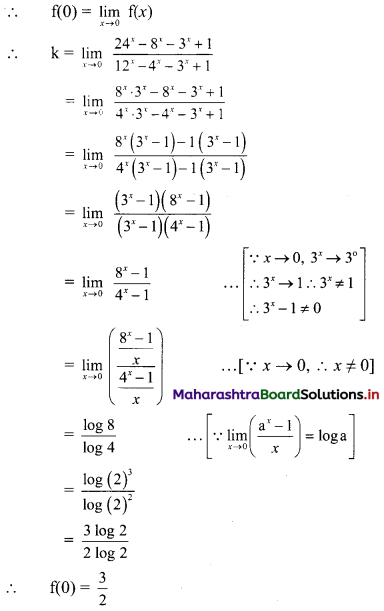(ii) If f(x) = $$\frac{5^{x}+5^{-x}-2}{x^{2}}$$, for x ≠ 0
= k for x = 0
is continuous at x = 0, find k.
Solution:
Function f is continuous at x = 0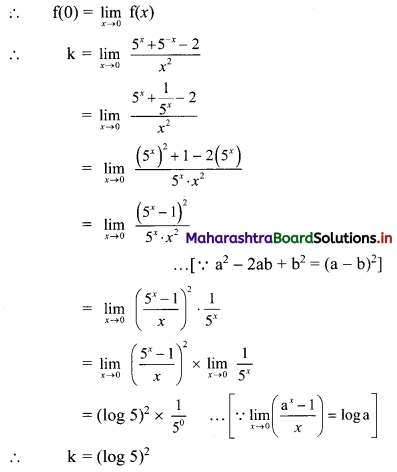(iii) For what values of a and b is the function
f(x) = ax + 2b + 18 for x ≤ 0
= x2 + 3a – b for 0 < x ≤ 2 = 8x – 2 for x > 2,
continuous for every x?
Solution:
Function f is continuous for every x.
∴ Function f is continuous at x = 0 and x = 2
As f is continuous at x = 0.
∴ $$\lim _{x \rightarrow 0^{-}} \mathrm{f}(x)=\lim _{x \rightarrow 0^{+}} \mathrm{f}(x)$$
∴ $$\lim _{x \rightarrow 0^{-}}(a x+2 b+18)=\lim _{x \rightarrow 0^{+}}\left(x^{2}+3 a-b\right)$$
∴ a(0) + 2b + 18 = (0)2 + 3a – b
∴ 3a – 3b = 18
∴ a – b = 6 …..(i)
Also, Function f is continous at x = 2
∴ $$\lim _{x \rightarrow 2^{-}} \mathrm{f}(x)=\lim _{x \rightarrow 2^{-}} \mathrm{f}(x)$$
∴ $$\lim _{x \rightarrow 2^{-}}\left(x^{2}+3 a-b\right)=\lim _{x \rightarrow 2^{-}}(8 x-2)$$
∴ (2)2 + 3a – b = 8(2) – 2
∴ 4 + 3a – b = 14
∴ 3a – b = 10 …..(ii)
Subtracting (i) from (ii), we get
2a = 4
∴ a = 2
Substituting a = 2 in (i), we get
2 – b = 6
∴ b = -4
∴ a = 2 and b = -4(iv) For what values of a and b is the function
f(x) = $$\frac{x^{2}-4}{x-2}$$ for x < 2
= ax2 – bx + 3 for 2 ≤ x < 3
= 2x – a + b for x ≥ 3
continuous in its domain.
Solution:
Function f is continuous for every x on R.
∴ Function f is continuous at x = 2 and x = 3.
As f is continuous at x = 2.
∴ $$\lim _{x \rightarrow 2^{-}} f(x)=\lim _{x \rightarrow 2^{+}} f(x)$$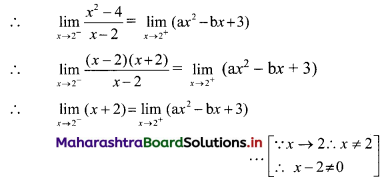∴ 2 + 2 = a(2)2 – b(2) + 3
∴ 4 = 4a – 2b + 3
∴ 4a – 2b = 1 …..(i)
Also function f is continuous at x = 3
∴ $$\lim _{x \rightarrow 3^{-}} f(x)=\lim _{x \rightarrow 3^{+}} f(x)$$
∴ $$\lim _{x \rightarrow 3^{-}}\left(a x^{2}-b x+3\right)=\lim _{x \rightarrow 3^{+}}(2 x-a+b)$$
∴ a(3)2 – b(3) + 3 = 2(3) – a + b
∴ 9a – 3b + 3 = 6 – a + b
∴ 10a – 4b = 3 …..(ii)
Multiplying (i) by 2, we get
8a – 4b = 2 …..(iii)
Subtracting (iii) from (ii), we get
2a = 1
∴ a = $$\frac{1}{2}$$
Substituting a = $$\frac{1}{2}$$ in (i), we get
4($$\frac{1}{2}$$) – 2b = 1
∴ 2 – 2b = 1
∴ 1 = 2b
∴ b = $$\frac{1}{2}$$
∴ a = $$\frac{1}{2}$$ and b = $$\frac{1}{2}$$